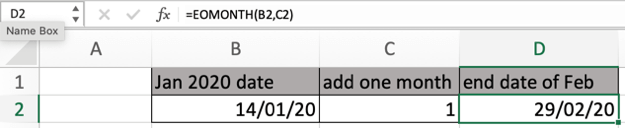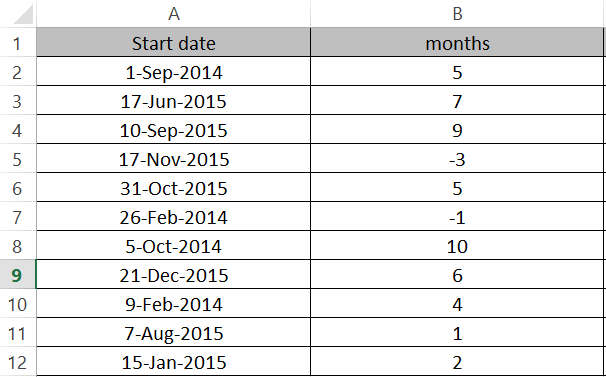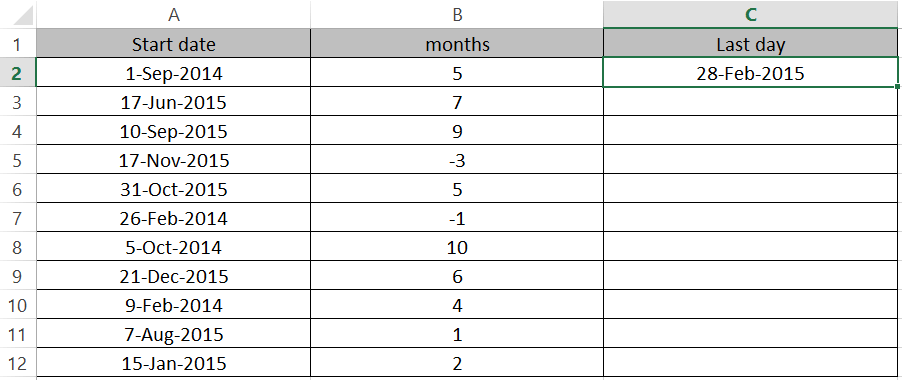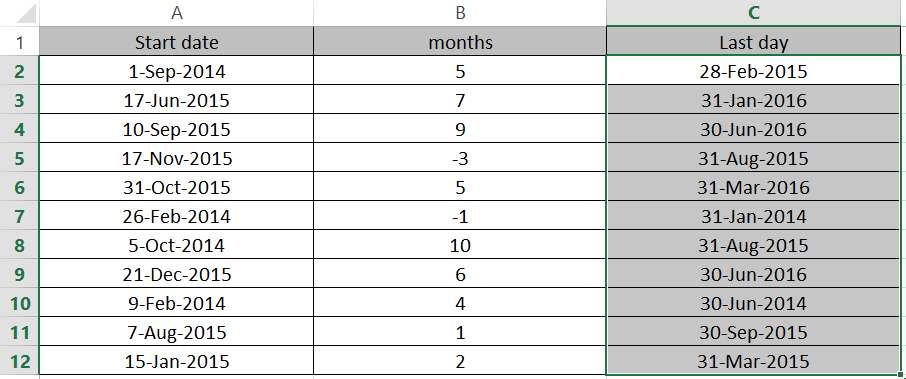# How to calculate the date at the end of a month in ExcelIn this article, we will learn How to calculate the date at the end of a month in Excel.

Scenario:

At times, we need to know the expiry date of any product given its period of expiry and manufactured date. And you would be thinking why to do it manually. Then YES. You are right. The Excel function does your work here. Let's understand how excel works with dates to get the end date for the month using the Excel EOMONTH function.

EOMONTH function in Excel

EOMONTH function is used to find the last day of the given month. It requires two things:

1. From the date you wish to count or the manufacturing date.
2. Number of months from the date or its living period.

EOMONTH function Syntax:

 =EOMONTH( Start_date, months)

start_date : a valid date representing the start date for the function.

Months : a positive or negative number to get the date for after or before the start date.

Example :

All of these might be confusing to understand. Let's understand how to use the function using an example. Here we have start dates in the first columns and period of months in the second column.Using the formula in the cell where we want the last day of the given month.

Formula:

 =EOMONTH(A2,B2)

Here arguments to the function are given as cell reference.To get the 5 months after the 1st September 2014. What is the last day of that month? The month comes out to be february and the last day of feb 2015 is 28th of Feb.

Copy the formula in other cells, select the cells taking the first cell where the formula is already applied, use the Ctrl + D shortcut key.As you can see from the above example, you can find the last day of any month using the EOMONTH function.

Sometimes the value returned by the function is a 5 digit number seems like some code digit or something. It's nothing, As Excel stores date and time values as serial numbers. So just change the format of the cell from General to Date. Or Select the type of date format from Custom Format cell box.

Here are some observational notes using the above function in Excel.

Notes:

1. Excel records dates as serial numbers for calculation purposes. So valid date format or else the function returns the #NUM! Error.
2. You can add or subtract months to get to the required month and then get the last date of the month.
3. The start_date plus the months yields a invaild date, the function returns the #NUM! error.

Hope this article about How to calculate the date at the end of a month in Excel is explanatory. Find more articles on calculating values and related Excel formulas here. If you liked our blogs, share it with your friends on Facebook. And also you can follow us on Twitter and Facebook. We would love to hear from you, do let us know how we can improve, complement or innovate our work and make it better for you. Write to us at info@exceltip.com.

Related Articles :

Count holidays between dates in Excel : count non working days between the two given dates using the DATEDIF and NETWORKDAYS function in Excel.

SUM price by weekdays in Excel : sum price values corresponding to the number of dates in a particular weekday using the SUMPRODUCT and WEEKDAY function in Excel.

How to Convert date to text in Excel : In this article we learned how to convert text into date, but how do you convert an excel date into text. To convert an excel date into text we have a few techniques.

Extract days between dates ignoring years in Excel : count the days between the two dates using different functions and mathematical operation in Excel

How to Convert date to text in Excel : In this article we learned how to convert text into date, but how do you convert an excel date into text. To convert an excel date into text we have a few techniques.

How to use the WORKDAYS function in Excel : Returns a workday date value after days added to the start_date using the WORKDAYS function in Excel.

Count Birth Dates By Month in Excel : count the number of dates lying in a particular month using the SUMPRODUCT and MONTH function in Excel.

Popular Articles :